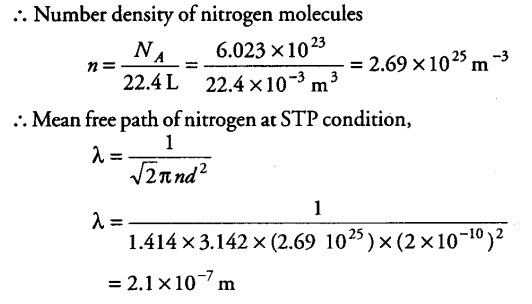# What will be the mean free path of nitrogen gas at STP

What will be the mean free path of nitrogen gas at STP of given diameter of nitrogen molecule = 2 A]

Given,
Diameter of oxygen molecule d = 2 A = 2 x {{10}^{-10}} m
At STP one mole of gas (or 22.4 L) of gas have
{ N }_{ A } = 6.023 x {{10}^{23}} molecules